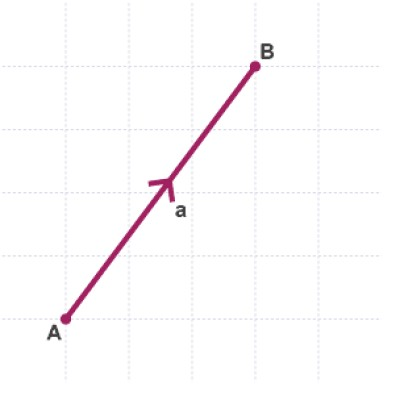List of public pages created with Protopage

# Aaron Varrow

## Plain sticky notes

1.Im one of the oldest in my year 2.I went to Kelvedon ST Marys Academy 3.I like maths

# Probability

## Plain sticky notes

### Sticky note

probability is likely hood between 0 and 1 e.g the probabilityof it raining tomorrow is 0.5/0.5

# Trapezoid

## Plain sticky notes

### Sticky note

In Euclidean geometry, a convex quadrilateral with at least one pair of parallel sides is referred to as a trapezoid in American English and as a trapezium in English outside North America. The parallel sides are called the bases of the trapezoid and the other two sides are called the legs or the lateral sides (if they are not parallel; otherwise there are two pairs of bases). A scalene trapezoid is a trapezoid with no sides of equal measure, in contrast to the special cases below. A trapezoid with vertices ABCD is denoted Trapezoid small icon.png ABCD. There is some disagreement whether parallelograms, which have two pairs of parallel sides, should be counted as trapezoids. Some define a trapezoid as a quadrilateral having exactly one pair of parallel sides (the exclusive definition), thereby excluding parallelograms. Others define a trapezoid as a quadrilateral with at least one pair of parallel sides (the inclusive definition), making the parallelogram a special type of trapezoid. The latter definition is consistent with its uses in higher mathematics such as calculus. The former definition would make such concepts as the trapezoidal approximation to a definite integral ill-defined. This article uses the inclusive definition and considers parallelograms as special cases of a trapezoid. This is also advocated in the taxonomy of quadrilaterals.

## Photos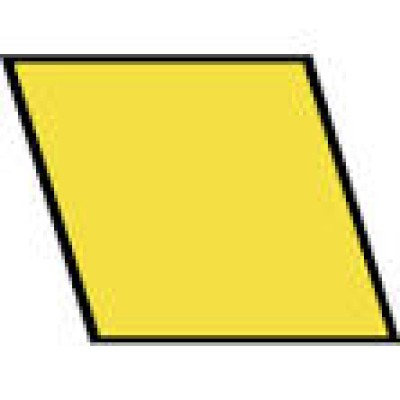# Substatution

## Plain sticky notes

### Sticky note

In mathematics, a change of variables is a basic technique used to simplify problems in which the original variables are replaced with other variables derived from the originals; the new and old variables being related in some specified way. The intent is that when expressed in new variables, the problem may become simpler, or equivalent to a better understood problem. A very simple example of a useful variable change can be seen in the problem of finding the roots of the sixth order polynomial: x^6 - 9 x^3 + 8 = 0. \, Sixth order polynomial equations are generally impossible to solve in terms of elementary functions. This particular equation, however, may be simplified by defining a new variable x3 = u. Substituting this into the polynomial: u^2 - 9 u + 8 = 0 \, which is just a quadratic equation with solutions: u = 1 \quad \text{and} \quad u = 8. The solution in terms of the original variable is obtained by replacing the original variable: x^3 = 1 \quad \text{and} \quad x^3 = 8 \quad \Rightarrow \qquad x = (1)^{1/3} = 1 \quad \text{and} \quad x = (8)^{1/3} = 2.\,

# Y = Mx + C and Graph

## Plain sticky notes

### Sticky note

y = mx + c We have seen graphs in the form y = mx + c before. When a graph is written in the form y = mx + c, m represents the gradient and c represents the y intercept. Take the example of the cost of a taxi ride at £1 + £3 per mile. In this case the gradient is the cost per mile and the intercept the £1 standing charge. This can be written as y = 3x + 1 This line has a gradient of 3 and a y intercept of 1.

## Photos

### y = mx + c

this here is a y=mx+c graph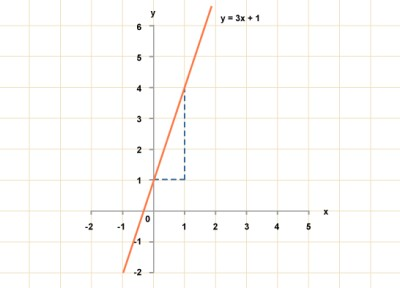# Scatter Graph

## Plain sticky notes

### scatter graph

A class took two tests. Test A was given early in the course and test B towards the end. The comparative results of these tests are given in the scatter graph below. Scatter graph 1 Each symbol on the graph shows the scores achieved in both tests by each of the pupils. So, for example, pupil A scored 60% on test A but only 20% on test B. Pupil C achieved the top mark in test A. Pupil P achieved the top mark in test B. Pupil marked D came second in both tests.

## Photos

### scatter graph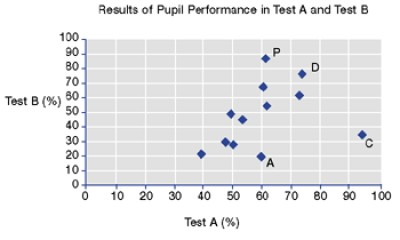# Parellel Lines

## Plain sticky notes

### Sticky note

parallel lines are lines in a plane which do not meet. The assumed existence and properties of parallel lines are the basis of Euclid's parallel postulate. Two lines in a plane that do not intersect or touch at any point are said to be parallel. By extension, a line and a plane, or two planes, in three-dimensional Euclidean space that do not share a point are said to be parallel.

## Photos

### parallel lines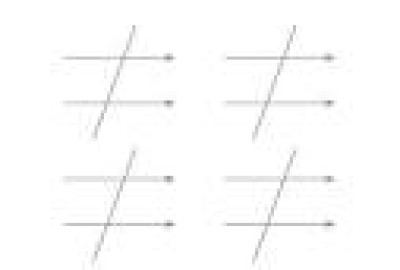# Transformations

## Photos

### mathematical enlargement

To enlarge the triangle with a scale factor of 2 and centre of enlargement 0, Points A1, B1 and C1 are connected to form an enlarged triangle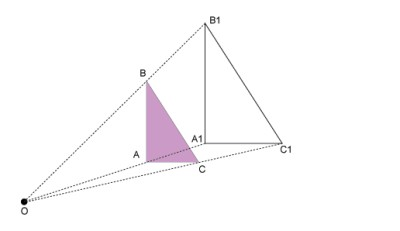### rotation

If you put a piece of paper on a table and place your pen in the middle of it, you can rotate the paper whilst keeping the pen in a fixed position. In doing this you are rotating the paper about its centre. 270 degree rotation, a three quarter turn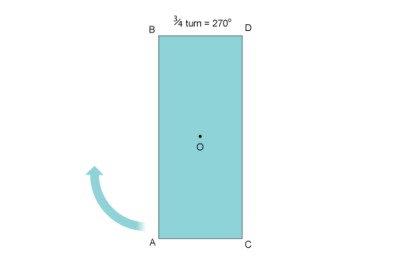### reflection

If you look in a mirror, you see your own image. You (the object) and your image appear to be the same distance from the mirror. An object and its image are always the same perpendicular distance from the mirror line. (Perpendicular means 'at right-angles to'.) Look at the following examples: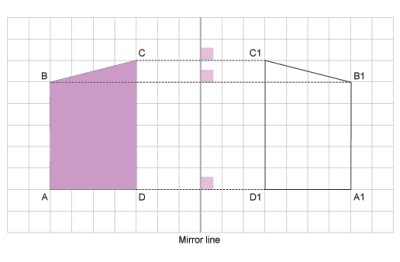# Solving Equations

## Plain sticky notes

### Sticky note

5x + 8 = 108 x = 20 108-8 = 100 100÷ 5 = 20

# Fractions

## Plain sticky notes

### Sticky note

Fraction can be converted to 2 othe things, such as decimal places and percentages. a ½ = 50% and in decimals it = 0.5

## Photos

### fractions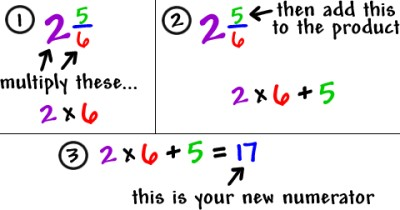# Percentages

## Plain sticky notes

### How to convert percentages to fractions

Step 1: Write down the percent divided by 100. Step 2: If the percent is not a whole number, then multiply both top and bottom by 10 for every number after the decimal point. (For example, if there is one number after the decimal, then use 10, if there are two then use 100, etc.) Step 3: Simplify (or reduce) the fraction

## Photos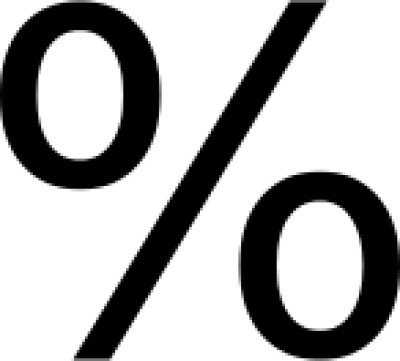# Circles and π(pi)

## Plain sticky notes

### Sticky note

A circle is a plane figure bounded by one line, called the circumference, such that all straight lines drawn from the center to the circumference are equal to one another. A straight line from the center to the circumference is a called a radius. A diameter is a straight line through the center and terminating in both directions on the circumference. A radius, then, is half of a diameter; equivalently, a diameter is twice a radius: To figure out the area of a circle you the radius^2 x Pi And to work out the circumference you do Diameter x pi

## Photos

### circle and the use of pi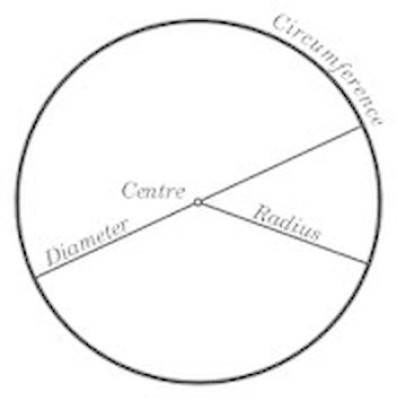# N'th Term

## Plain sticky notes

### Sticky note

Linear sequences 1, 4, 7, 10 is a sequence starting with 1. You get the next term by adding 3 to the previous term. You are often asked to find a formula for the nth term. linear sequence: 1, 4, 7, 10 As the common difference is 3, try 3n. When n = 1, 3n = 3, and we subtract 2 to make the first term correct. So the nth term = 3n - 2 This method will always work for sequences where the difference between terms stays the same.

# Standard form

## Plain sticky notes

### Sticky note

Standard form is making a number that is a decimal x 10 to the power of a number for example 1.2 x 10² = 120 to make a number in standaed form you need to put the decimal point infront of the first non-zero number 3400 = 3.4 x 10³

## Photos

### Standard form in a picture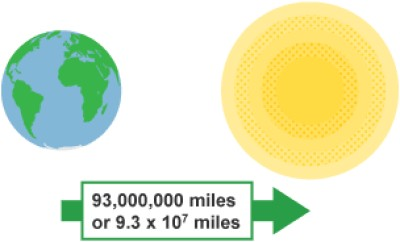# Ratio

## Plain sticky notes

### Sticky note

if i had ratio 3:5 and i had to sperate it over 72 i would add the 3 and 5 to 8 and figure that 8 goes in 72, 9 times. Therefore i times both the numbers by 9 to get 27:45

# Trigonometry

## Plain sticky notes

### The Formula

Easiest way to remember, SoH CaH ToA Sinx x Opposite = Hypotenuse Cosx x Adjacent = Hypotenuse Tanx x Opposite = Adjacent Hypotenuse: The longest side. Ajacent: Next to the angle Opposite: Opposite the angle you have been given or trying to find out. if you were trying to find out the angle you would do sine to the power of -1

## Photos

### Trigonometry

On this diagram the angle would be on the bottom left, because of the side on the right being the opposite.# Stratified Sampling

## Plain sticky notes

### Stratified Sampling

Stratified sampling refers to a type of sampling method . With stratified sampling, the researcher divides the population into separate groups, called strata. Then, a probability sample (often a simple random sample ) is drawn from each group. Stratified sampling has several advantages over simple random sampling. For example, using stratified sampling, it may be possible to reduce the sample size required to achieve a given precision. Or it may be possible to increase the precision with the same sample size.

# Bisecting Angles

## Plain sticky notes

### Perpendicular Bisector of a Line Segment

The locus of a point which moves so that it is an equal distance from two points, A and B, is the perpendicular bisector of the line joining A and B. Perpendicular means at right angles to. Bisector means cuts in half. Draw a horizontal line with an acute angle. Put the point of your compass on X (the left side of the horizontal line) and put the pencil over half way and draw an arc. Without adjusting the compass, put it on point Y (the right side of the line) and draw another arc. Label the points where they cross, A and B, and draw a line through these points and this is perpendicular bisector. REMEMBER DON'T RUB OUT THE CONSTRUCTION LINES

### Bisecting Angles

O is the vertex of the angle we want to bisect. Place your compass on O and draw an arc that crosses both sides of the angle. Label the crossing points A and C. Place your compass on A and draw an arc between the two sides of the angle Without adjusting your compass place it on C and draw another arc that cuts the one you just drew. Label the point where they cross B. Draw a straight line through O and B. REMEMBER DON'T RUB OUT THE CONSTRUCTION LINES

## Photos

### Bisecting Angles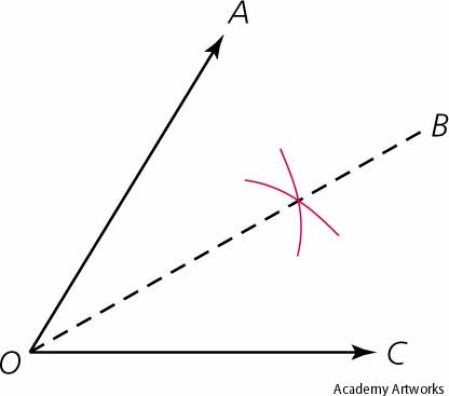### Perpendicular Bisector of a Line Segment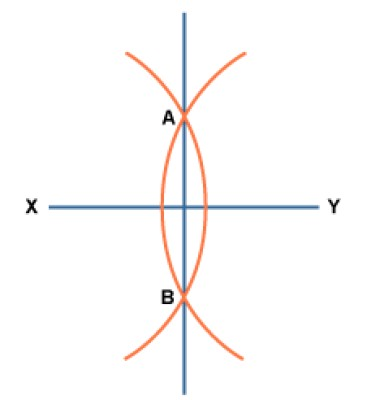# Simultaneous Equations

## Plain sticky notes

### Sticky note

Solve these simultaneous equations and find the values of x and y. Equation 1: 2x + y = 7 Equation 2: 3x - y = 8 Add the two equations to eliminate the ys: 2x + y = 7 3x - y = 8 ------------ 5x = 15 x = 3 Now you can put x = 3 in either of the equations. Substitute x = 3 into the equation 2x + y = 7: 6 + y = 7 y = 1 So the answers are x = 3 and y = 1 Sometimes you will need to multiply one of the equations before you can add or subtract.

# Vectors

## Plain sticky notes

### Sticky note

A vector describes a movement from one point to another. A vector quantity has both direction and magnitude (size). A scalar quantity has only magnitude. A vector can be represented by a line segment labelled with an arrow A vector between two points A and B is described as:

## Photos